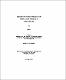## Mechanisms for platelet hyperactivity and abnormal calcium homeostasis in dia etes mellitusThe overall objective of this thesis is to determine the mechanisms responsible for platelet hyperactivity in diabetes mellitus. The first part of the study was designed to investigate whether platelet Ca$\sp{2+}$ homeostasis is deranged in diabetes and thus may be responsible for platelet hyperactivity. Platelets were obtained from a group of metabolically uncontrolled diabetic patients and from a group of normal healthy subjects. HbA$\rm\sb{1c}$ level was used as an index of chronic glycemic control. Both the basal cytosolic Ca$\sp{2+}$ concentration and agonist (thrombin and collagen)-stimulated $\rm\lbrack Ca\sp{2+}\rbrack\sb{i}$ responses were enhanced in platelets from diabetic patients. The contents of Ca$\sp{2+}$ in the platelet intracellular store (DTS) and the ability to release Ca$\sp{2+}$ from DTS after thrombin stimulation did not differ in these 2 groups of subjects. The sequestration or extrusion processes of cytosolic Ca$\sp{2+}$ were impaired in platelets from diabetics. The purpose of the second part of the study was to determine the possible mechanisms for abnormal platelet calcium homeostasis in diabetes, mainly focusing on the plasma membrane Na$\sp+$-Ca$\sp{2+}$ exchanger. The existence and physiological role of the Na$\sp+$-Ca$\sp{2+}$ exchanger in platelets from normal subjects was investigated. The specificity of the Na$\sp+$-Ca$\sp{2+}$ exchange blocker CBDMB (an amiloride analogue) in intact platelets was found to inhibit the Na$\sp+$-Ca$\sp{2+}$ exchanger, and have no effect on the Na$\sp+$-H$\sp+$ exchanger at $\mu$M concentrations. In platelets from normal subjects, the Na$\sp+$-Ca$\sp{2+}$ exchanger works in the forward mode in the resting state and after thrombin stimulation, however following collagen stimulation the Na$\sp+$-Ca$\sp{2+}$ exchanger works in the reverse mode. In the second series of experiments, sensitivity to CBDMB was utilized to study the direction and activity of the Na$\sp+$-Ca$\sp{2+}$ exchanger in intact platelets. In normal subjects, CBDMB increased resting $\rm\lbrack Ca\sp{2+}\rbrack\sb{i}$ whereas in diabetes, CBDMB had no effect on resting $\rm\lbrack Ca\sp{2+}\rbrack\sb{i}.$ After thrombin activation, CBDMB increased the second phase of the thrombin-induced $\rm\lbrack Ca\sp{2+}\rbrack\sb{i}$ response in normals. In platelets from diabetics, CBDMB decreased the thrombin-induced $\rm\lbrack Ca\sp{2+}\rbrack\sb{i}$ response. In platelets from normal subjects, CBDMB decreased the collagen-stimulated $\rm\lbrack Ca\sp{2+}\rbrack\sb{i}$ response. In diabetics the decrease in $\rm\lbrack Ca\sp{2+}\rbrack\sb{i}$ following CBDMB in collagen-activated platelets was enhanced. In diabetes the direction and activity of the Na$\sp+$-Ca$\sp{2+}$ exchanger are altered. The third part of the study evaluated the direct effect of hyperglycemia on platelets in vitro. High glucose (43 mM) had no acute effect on thrombin and collagen-induced increases in $\rm\lbrack Ca\sp{2+}\rbrack\sb{i}$ or on aggregation. When the PRP (platelet rich plasma) was incubated in high glucose at 37$\sp\circ$C for 24 hrs, several differences were observed when compared to platelets incubated in 5 mM Glucose. PRP with EDTA as the anticoagulant, was divided into 3 groups: control (5 mM D-glucose medium); high mannitol (5mM D-Glucose + 40 mM Mannitol, as an osmotic control) and high glucose (45mM D-Glucose). In the high glucose group the first peak and second phase of thrombin-induced $\rm\lbrack Ca\sp{2+}\rbrack\sb{i}$ were increased. The enhanced second phase was inhibited by CBDMB. In addition, the collagen-stimulated $\rm\lbrack Ca\sp{2+}\rbrack\sb{i}$ response and aggregation were increased in the high glucose group. (Abstract shortened by UMI.)